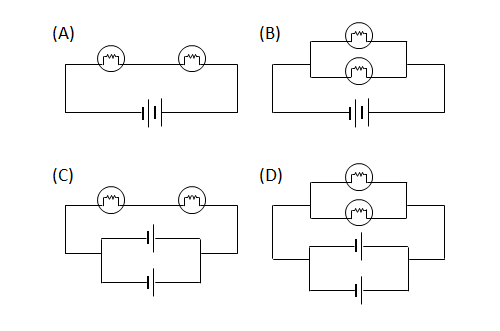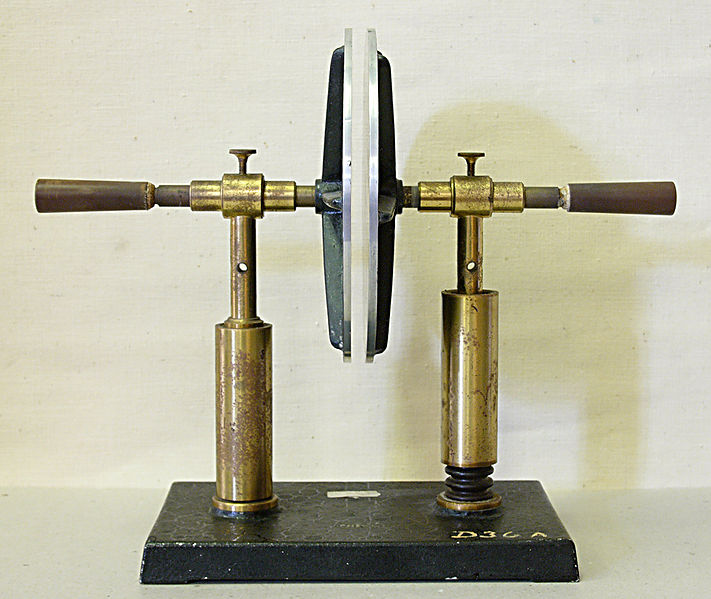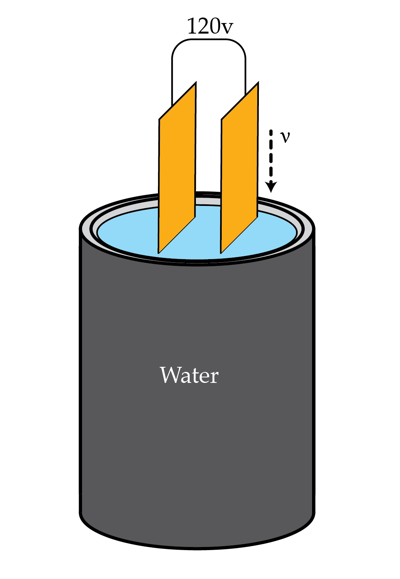Electricity and Magnetism

# Circuit Behavior: Level 2-3 Challenges

A technician has 10 resistors each of resistance $$0.1 \Omega$$. Find the largest and smallest resistance that he can obtain by combining these resistors.Find $$V_A$$ in volts.Bill has two identical lamps each of resistance 5 ohms and two 9-volt-batteries. If he wants to light the two lamps for a duration as long as possible, how should he connect the lamps and batteries?When a charged capacitor is disconnected from its source it will eventually discharge. This is because a small amount of charge leaks through the dielectric between the plates of the capacitor. Suppose we fill a parallel plate capacitor with a ceramic of relative permittivity $$\epsilon=2.1$$ and resistivity $$\rho=1.4 \times 10^{13}~\Omega \cdot \mbox{m}$$. The capacitor is charged by connecting it to a voltage source. How long will it take in seconds for the capacitor to lose half of the charge acquired after disconnecting it from the source?

###### Image credit: Wikipedia

A 120V source is connected across the plates of a parallel-plate capacitor. The capacitor is being submerged vertically at constant speed into a container filled with water. What current in Amps flows through the voltage source during this process if it takes $$\tau=20 s$$ to totally submerge the capacitor? The capacitance (in air) of the capacitor is $$C_{0}=12\mu F$$ and water's relative permittivity is $\epsilon=81.$Hint: If the capacitor is partially submerged at an instant of time try figuring out how to treat it as two separate capacitors at that instant.

×